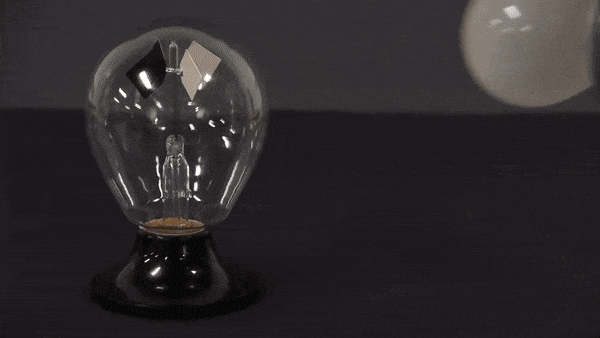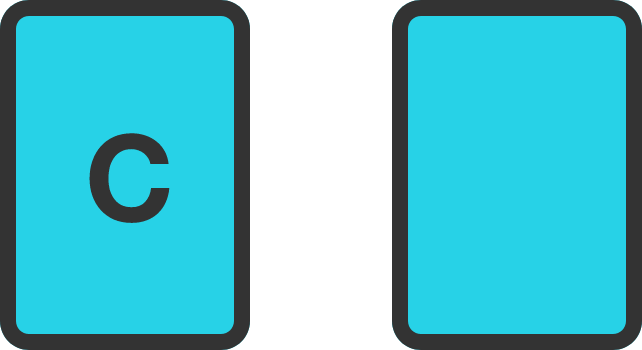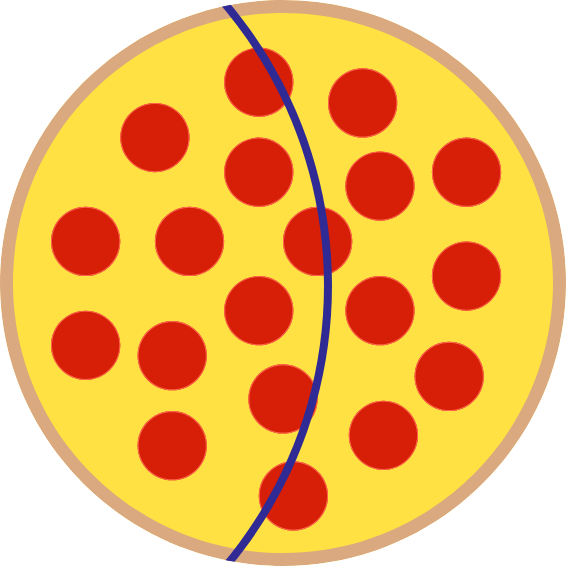# Problems of the Week

Contribute a problem

# 2018-03-05 BasicThese blades are white on one side and black on the other. They are placed in a glass chamber filled with gas at very low pressure.

Why does the lightbulb make the blades rotate?There are two cards—one with the letter "C" and the other blank.

You want to check if the following statement is true for the two cards:

If a card has a number on one side, then it is blank on the other.

Which card(s) must you flip?

If you reverse all the digits of a positive integer, is it ever possible for the resulting number to be twice the original number?

Hint: You can approach this like a cryptogram puzzle; with three digits you would solve $ABC + ABC = CBA$ where each letter represents a digit.

Note: The problem is done in base 10.I cut a pizza in the shape of a circular arc such that there are 2 pieces of equal area.

Is it possible that these 2 pieces have the same amount of crust on the circumference?

Assume the crust is 1-dimensional, i.e. it's just the circumference of the pizza with no area.

Take the product of all positive divisors of 100: $1 \times 2 \times 4 \times 5 \times \cdots \times 100 .$

This product is divisible by some $2^m ,$ where $m$ is an integer. What is the largest possible value of $m?$

×